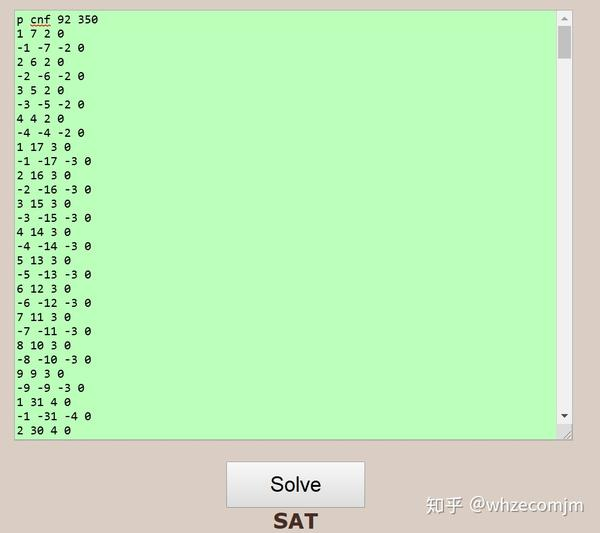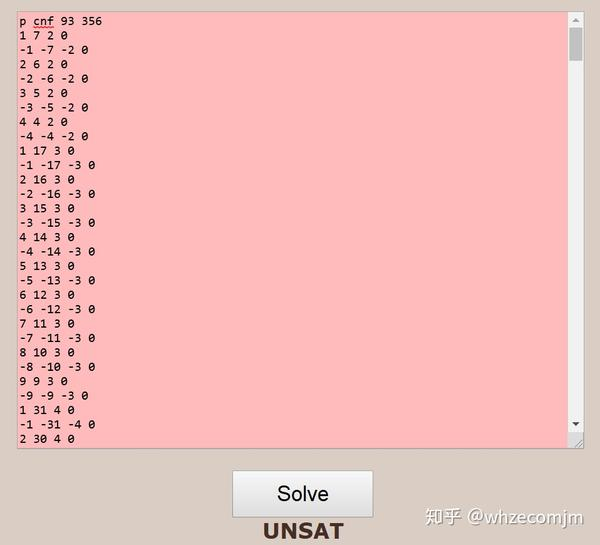## 方程的染色问题

### partition regular equations with Ramsey theory.

Definition 1. An equation $f(x,y,z,\dots)=0$ is partition regular (or simply regular) if, and only if, for any finite partition of $\mathbb{N}$ into $C_1, C_1,\dots, C_r$, there exists some $C_i$ containing a non-trivial solution to the equation.

Schur 最早研究了分割正规的Schur方程，也就是最简单的线性齐次方程 $x+y=z$。Schur定理表明，对自然数的任意有限染色，上述方程都有单色解。为了更好的理解，我们考虑二染色，用反证法可以证明在二染色情况下，总存在单色解。

Definition 2. Given an equation or a system of equations $\mathcal{L}$ and finite $r$ colors, the Rado number $R(\mathcal{L},r)$ is the least number such that any $r$-coloring of ${1,2,\dots,R(\mathcal{L},r)}$ must contain a monochromatic solution to $\mathcal{ L}$.

Theorem 3 (Rado’s Theorem ). A linear homogeneous equation $a_1 x_1+\cdots+a_nx_n=0$ is partition regular on $\mathbb{N}$ if and only if there exists a nonempty set $J\subseteq{1,\dots,n}$ such that $\sum_{j\in J}a_j=0$.

Definition 4. The degree of regularity of an equation or a system of equations $\mathcal{L}$ is the largest integer $r\geq 0$, if any, such that $\mathcal{L}$ is $r$-regular. This number is denoted by $\mathrm{dor}(\mathcal{L})$. In particular, if $\mathrm{dor}(\mathcal{L})=\infty$, then $\mathcal{L}$ is regular.

$$R(x+y=2z^2,2)=93.$$ 下面附上生成 cpf 的python 代码（几年没写过代码）和结果：

  1 2 3 4 5 6 7 8 9 10 11 12 13 14 15 16 17 18  n=94; # then 93 # get the number of clauses for any n<100. def clauses(n,k=0): for j in range(2,10): for i in range(1,j**2+1): if iReferences.

 R. Rado, Studien zur Kombinatorik, Math. Z. 36 (1933), 242–280.
 S. W. Golomb and L. D. Baumert. “Backtrack programming.” Journal of the ACM (JACM) 12.4 (1965): 516-524.
 M. Heule, Schur number five. Thirty-Second AAAI Conference on Artificial Intelligence. 2018.
 M. Heule, O. Kullmann, and V. Marek, Solving and verifying the boolean Pythagorean triples problem via cube-and-conquer. International Conference on Theory and Applications of Satisfiability Testing. Springer, Cham, 2016.
 M. Nasso and L. Baglini. Ramsey properties of nonlinear Diophantine equations. Advances in Mathematics 324 (2018): 84-117.
 B. Green and S. Lindqvist. Monochromatic Solutions to x+y=z2. Canadian Journal of Mathematics 71.3 (2019): 579-605.

Built with Hugo
Theme Stack designed by Jimmy